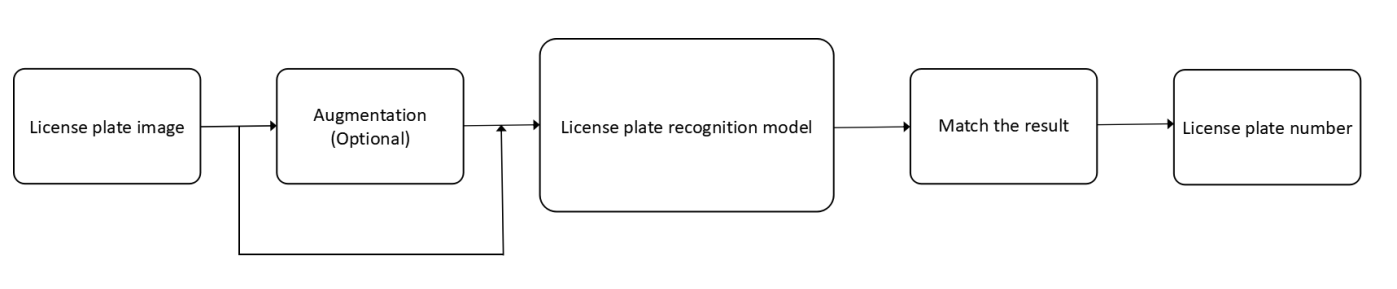# License Plate Recognition with OpenVINO™¶

This tutorial is also available as a Jupyter notebook that can be cloned directly from GitHub. See the installation guide for instructions to run this tutorial locally on Windows, Linux or macOS. To run without installing anything, click the launch binder button.

This notebook demonstrates license plate recognition with OpenVINO, using the License Plate Recognition Model from Open Model Zoo. This model uses a small-footprint network trained end-to-end to recognize Chinese license plates in traffic.

License plate recognition model helps you get the Chinese license plate number precisely in no time. The input of the color license plate image can be of any size. It will be resized and augmented before being put into the model. After matching the result to correct character, you can get the license plate number. The notebook shows how to create the following pipeline:flowchart.png

Note: Augmentation method on image is optional and it may lead to the wrong recognition result. Therefore, it is recommended to use it under special conditions, such as image being overexposed or too dark.

Example image data comes from CCPD (Chinese City Parking Dataset, ECCV)

## Imports¶

import cv2
import matplotlib
import matplotlib.pyplot as plt
import numpy as np
from openvino.runtime import Core


## The Model¶

Use the omz_downloader tool from the openvino-dev package to download the selected model. If the model is already downloaded, it will be retrieved from the cache.

# A directory where the model will be downloaded.
base_model_dir = "model"

# The name of the model from Open Model Zoo.

# Selected precision (FP32, FP16, FP16-INT8).
precision = "FP16"

f"--name {model_name} "
f"--precision {precision} "
f"--output_dir {base_model_dir} "
f"--cache_dir {base_model_dir}"
)

# This code is provided for the first download of the model.

################|| Downloading license-plate-recognition-barrier-0001 ||################



First, initialize OpenVINO Runtime. Then, read the network architecture and model weights from the .bin and .xml files to compile for the desired device. You can choose manually CPU, GPU, MYRIAD etc.

If you want OpenVINO to decide which hardware offers the best performance, you need to use AUTO.

# The output path for the conversion
converted_model_path = f"model/intel/{model_name}/{precision}/{model_name}.xml"

# Initialize OpenVINO Runtime
ie_core = Core()
# Read the network and corresponding weights from a file.
# Compile the model for the CPU (you can choose manually CPU, GPU, MYRIAD etc.)
# or let the engine choose the best available device (AUTO).
compiled_model = ie_core.compile_model(model=model, device_name="CPU")

# Get input and output nodes.
input_layer = compiled_model.input(0)
output_layer = compiled_model.output(0)

# Get the input size.
input_height, input_width = list(input_layer.shape)[2:4]


## Inference¶

### Using ImageLoader to Process Image¶

Model Limitations: Only “blue” license plates, which are common in public, were tested thoroughly. Other types of license plates may underperform.

#### Model Input:¶

name: “data” , shape: [1x3x24x94] - An input image in following format [1xCxHxW]. Expected color order is BGR.

name: “seq_ind” , shape: [88, 1] - An auxiliary blob that is needed for correct decoding. Set this to [1, 1, ..., 1].

Notes: Since the license plate image could be of any size, use ImageLoader to resize it to fit the model input requirements.

def show_image(
origin_image: np.ndarray, input_image: np.ndarray
) -> matplotlib.figure.Figure:
"""
Visualise how the image we processed.

:param origin_image: Any size of color image.
:param input_image: The input of the model before dimension transposed and expanded.
:returns: Matplotlib figure.
"""
figure, axis = plt.subplots(1, 2, figsize=(18, 9), squeeze=False)

# Adjust the image channels to the correct order.
origin_image = cv2.cvtColor(origin_image, cv2.COLOR_BGR2RGB)
axis[0, 0].imshow(origin_image)
axis[0, 0].set_title("Source Image")

# Adjust the image channels to the correct order.
input_image = cv2.cvtColor(input_image, cv2.COLOR_BGR2RGB)
axis[0, 1].imshow(input_image)
axis[0, 1].set_title("Input Image")

return figure

def image_loader(image_path: str, augmentation: str = "None") -> np.ndarray:
"""
Process the image if neccessary and then
resize its shape to fit the input requirements.

:param image_path: Relative storage path of color image.
:param augmentation: Image augmentation method, if 'None', do nothing.
Including 'None', 'Laplace' and 'EquaHist'.
:returns: The input of the model. The dimension is 1x3x24x94.

"""

# If a gray image passed in, the program will stop.
assert len(ori_img.shape) == 3, "Failed to load image."
print(f"Origin image shape: {ori_img.shape}")

# If an invalid augmentation method passed in, the program will stop.
assert augmentation in [
"None",
"Laplace",
"EquaHist"
], "Invalid Augmentation."

if augmentation == "None":
img = ori_img

elif augmentation == "Laplace":
kernel_sharpen = np.array([[0, 1, 0], [1, -4, 1], [0, 1, 0]])
img = cv2.filter2D(ori_img, -1, kernel_sharpen)

elif augmentation == "EquaHist":
img0 = cv2.equalizeHist(ori_img[:, :, 0])
img1 = cv2.equalizeHist(ori_img[:, :, 1])
img2 = cv2.equalizeHist(ori_img[:, :, 2])

img = cv2.merge([img0, img1, img2])

# Resize its shape to fit the model input requirements.
resized_img = cv2.resize(img, (input_width, input_height))

# Visualize the way the image is processed.
show_image(origin_image=ori_img, input_image=resized_img)
trans_img = resized_img.transpose(2, 0, 1)
input_img = np.expand_dims(trans_img, axis=0)
return input_img


### Do the Inference¶

Match the output array into correct recognition results. Run license plate recognition model with the input and then match the result.

#### Process the Image¶

# The path of the test license plate image.
test_image_path = "data/example.png"


Origin image shape: (96, 181, 3)#### Input the Data and Get the Result¶

# An auxiliary blob that is needed for correct decoding.
# Set this to [1, 1, ..., 1].
auxiliary_blob = np.array( * 88)
# Resize it to fit the shape: [88, 1].
auxiliary_blob = np.resize(auxiliary_blob, (88, 1))

# Get the result.
result = compiled_model([input_img, auxiliary_blob])[output_layer]


#### Match the Output Array into Correct Recognition Results¶

def result_to_string(result: np.ndarray) -> str:
"""
Match the output array into correct recognition results.

:param result: The output of the model. The dimension is 1x88x1x1.
:returns: The license plate recognition results.
"""

# Each float is an integer number encoding a character according to this dictionary.